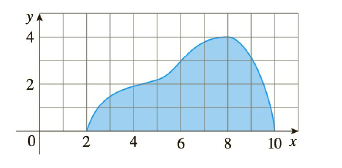Chapter 5.2, Problem 45E

Chapter
Section
Textbook Problem

# (a) If the region shown in the figure is rotated about the x-axis to form a solid, use the Midpoint Rule with n = 4 to estimate the volume of the solid.(b) Estimate the volume if the region is rotated about the y-axis. Again use the Midpoint Rule with n = 4.

To determine

Part (a):

To estimate:

The volume of the solid forthe region shown in the figure is rotated about the x axis

Explanation

1) Concept:

i. If the cross section is a disc and the radius of the disc is in terms of x  or y, then area  A=π radius2

ii. The volume of the solid revolution about the x-axis is V= abA(x)dx

iii. Use midpoint rule to evaluate the integral.

Midpoint Rule:

abf(x)dx=Mni=1nfxi-x=x[fxi-+ .+ fxn-]

where  x=b - an, n is the number of subintervals and  xi-=12xi-1+xi is midpoint of [xi-1, xi]

2) Given:

n= 4

3) Calculation:

The region rotates about the x– axis, so the cross-section is perpendicular to the x-axis.

From the graph, the region lies between x= 2 to x=10

And n=4

So the subintervals are, 2, 4, 4, 6, 6, 8, 8, 10

Thus, x1-=3, x2-=5, x3-=7, x4-=9  are the midpoints of the above subintervals

To determine

Part (b):

To estimate:

The volume of the solid for the region shown in the figure is rotated about the y axis

### Still sussing out bartleby?

Check out a sample textbook solution.

See a sample solution

#### The Solution to Your Study Problems

Bartleby provides explanations to thousands of textbook problems written by our experts, many with advanced degrees!

Get Started

#### In Exercises 41-48, find the indicated limit given that limxaf(x)=3 and limxag(x)=4 45. limxag(x)

Applied Calculus for the Managerial, Life, and Social Sciences: A Brief Approach

#### 6. Find the maximum value of the function subject to .

Mathematical Applications for the Management, Life, and Social Sciences

#### Evaluate the integral. 9. 24x+2x2+3x4dx

Single Variable Calculus: Early Transcendentals

#### π does not exist

Study Guide for Stewart's Single Variable Calculus: Early Transcendentals, 8th